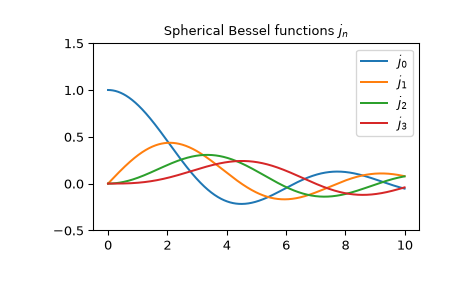# scipy.special.spherical_jn¶

scipy.special.spherical_jn(n, z, derivative=False)[source]

Spherical Bessel function of the first kind or its derivative.

Defined as ,

$j_n(z) = \sqrt{\frac{\pi}{2z}} J_{n + 1/2}(z),$

where $$J_n$$ is the Bessel function of the first kind.

Parameters
nint, array_like

Order of the Bessel function (n >= 0).

zcomplex or float, array_like

Argument of the Bessel function.

derivativebool, optional

If True, the value of the derivative (rather than the function itself) is returned.

Returns
jnndarray

Notes

For real arguments greater than the order, the function is computed using the ascending recurrence . For small real or complex arguments, the definitional relation to the cylindrical Bessel function of the first kind is used.

The derivative is computed using the relations ,

\begin{align}\begin{aligned}j_n'(z) = j_{n-1}(z) - \frac{n + 1}{z} j_n(z).\\j_0'(z) = -j_1(z)\end{aligned}\end{align}

New in version 0.18.0.

References

1

https://dlmf.nist.gov/10.47.E3

2

https://dlmf.nist.gov/10.51.E1

3

https://dlmf.nist.gov/10.51.E2

AS

Milton Abramowitz and Irene A. Stegun, eds. Handbook of Mathematical Functions with Formulas, Graphs, and Mathematical Tables. New York: Dover, 1972.

Examples

The spherical Bessel functions of the first kind $$j_n$$ accept both real and complex second argument. They can return a complex type:

>>> from scipy.special import spherical_jn
>>> spherical_jn(0, 3+5j)
(-9.878987731663194-8.021894345786002j)
>>> type(spherical_jn(0, 3+5j))
<class 'numpy.complex128'>


We can verify the relation for the derivative from the Notes for $$n=3$$ in the interval $$[1, 2]$$:

>>> from scipy.special import spherical_jn
>>> x = np.arange(1.0, 2.0, 0.01)
>>> np.allclose(spherical_jn(3, x, True),
...             spherical_jn(2, x) - 4/x * spherical_jn(3, x))
True


The first few $$j_n$$ with real argument:

>>> import matplotlib.pyplot as plt
>>> from scipy.special import spherical_jn
>>> x = np.arange(0.0, 10.0, 0.01)
>>> fig, ax = plt.subplots()
>>> ax.set_ylim(-0.5, 1.5)
>>> ax.set_title(r'Spherical Bessel functions $j_n$')
>>> for n in np.arange(0, 4):
...     ax.plot(x, spherical_jn(n, x), label=rf'$j_{n}$')
>>> plt.legend(loc='best')
>>> plt.show()